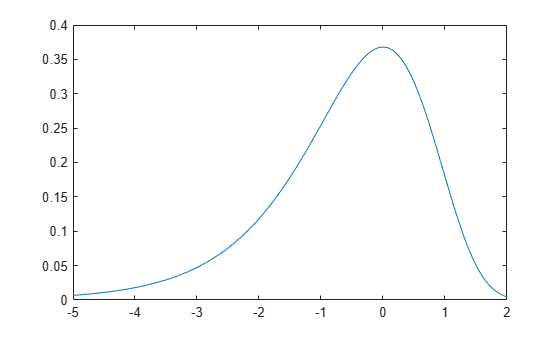Documentation

### This is machine translation

Mouseover text to see original. Click the button below to return to the English version of the page.

## Extreme Value Distribution

### Definition

The probability density function for the extreme value distribution with location parameter µ and scale parameter σ is

`$y=f\left(x|\mu ,\sigma \right)={\sigma }^{-1}\mathrm{exp}\left(\frac{x-\mu }{\sigma }\right)\mathrm{exp}\left(-\mathrm{exp}\left(\frac{x-\mu }{\sigma }\right)\right)$`

This form of the probability density function is suitable for modeling the minimum value. To model the maximum value, use the negative of the original values.

If T has a Weibull distribution with parameters a and b, then log T has an extreme value distribution with parameters µ = log a and σ = 1/b.

### Background

Extreme value distributions are often used to model the smallest or largest value among a large set of independent, identically distributed random values representing measurements or observations. The extreme value distribution is appropriate for modeling the smallest value from a distribution whose tails decay exponentially fast, such as, the normal distribution. It can also model the largest value from a distribution, such as the normal or exponential distributions, by using the negative of the original values.

For example, the following fits an extreme value distribution to minimum values taken over 1000 sets of 500 observations from a normal distribution.

```rng default; % For reproducibility xMinima = min(randn(1000,500), [], 2); paramEstsMinima = evfit(xMinima); y = linspace(-5,-1.5,1001); histogram(xMinima,-4.75:.25:-1.75); p = evpdf(y,paramEstsMinima(1),paramEstsMinima(2)); line(y,.25*length(xMinima)*p,'color','r')```The following fits an extreme value distribution to the maximum values in each set of observations.

```rng default; % For reproducibility xMaxima = max(randn(1000,500), [], 2); paramEstsMaxima = evfit(-xMaxima); y = linspace(1.5,5,1001); histogram(xMaxima,1.75:.25:4.75); p = evpdf(-y,paramEstsMaxima(1),paramEstsMaxima(2)); line(y,.25*length(xMaxima)*p,'color','r')```Although the extreme value distribution is most often used as a model for extreme values, you can also use it as a model for other types of continuous data. For example, extreme value distributions are closely related to the Weibull distribution. If `T` has a Weibull distribution, then `log(T)` has a type 1 extreme value distribution.

### Parameters

The function `evfit` returns the maximum likelihood estimates (MLEs) and confidence intervals for the parameters of the extreme value distribution. The following example shows how to fit some sample data using `evfit`, including estimates of the mean and variance from the fitted distribution.

Suppose you want to model the size of the smallest washer in each batch of 1000 from a manufacturing process. If you believe that the sizes are independent within and between each batch, you can fit an extreme value distribution to measurements of the minimum diameter from a series of eight experimental batches. The following code returns the MLEs of the distribution parameters as `parmhat` and the confidence intervals as the columns of `parmci`.

```x = [19.774 20.141 19.44 20.511 21.377 19.003 19.66 18.83]; [parmhat, parmci] = evfit(x)```
```parmhat = 20.2506 0.8223 parmci = 19.644 0.49861 20.857 1.3562 ```

You can find mean and variance of the extreme value distribution with these parameters using the function `evstat`.

`[meanfit, varfit] = evstat(parmhat(1),parmhat(2))`
```meanfit = 19.776 varfit = 1.1123```

### Examples

#### Compute the Extreme Value Distribution pdf

Compute the pdf of an extreme value distribution.

```t = [-5:.01:2]; y = evpdf(t);```

Plot the pdf.

```figure; plot(t,y)```The extreme value distribution is skewed to the left, and its general shape remains the same for all parameter values. The location parameter, `mu`, shifts the distribution along the real line, and the scale parameter, `sigma`, expands or contracts the distribution.

The following plots the probability function for different combinations of `mu` and `sigma`.

```x = -15:.01:5; plot(x,evpdf(x,2,1),'-', ... x,evpdf(x,0,2),':', ... x,evpdf(x,-2,4),'-.'); legend({'mu = 2, sigma = 1', ... 'mu = 0, sigma = 2', ... 'mu = -2, sigma = 4'}, ... 'Location','NW') xlabel('x') ylabel('f(x|mu,sigma)')```